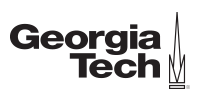Ir al contenido principal

# ​ Explore the fundamentals of linear algebraCertificación Profesional en
Introductory Linear Algebra
GTx

## Lo que aprenderás

• Evaluate mathematical expressions to compute quantities that deal with linear systems.
• Construct an LU factorization of a matrix and use it to solve linear systems of equations.
• Apply matrix algebra and linear transformations to solve and analyze problems in computer graphics and economics.
• Apply subspaces and invertibility to characterize and analyze matrices and solution sets of linear systems.

The first of the two courses will introduce systems of equations, which live at the heart of linear algebra. In this course you will explore fundamental concepts by exploring definitions and theorems that give a basis for this subject. You will apply an algorithm for solving linear systems that will be used for computations and for gaining insight into the properties of linear systems. This insight will all you to reduce problems involving linear combinations of vectors to approaches that involve systems of linear equations. You will also explore linear independence and linear transformations. They have an essential role throughout applications of linear algebra in many areas of industry, science, and engineering.

In the second of these two courses you will see how we can apply the Invertible Matrix Theorem to describe how a square matrix might be used to solve linear equations. This theorem is a fundamental role in linear algebra, as it synthesizes many of the concepts introduced in the first course into one succinct concept. You will then explore theorems and algorithms that will allow you to apply linear algebra in ways that involve two or more matrices. You will examine partitioned matrices and matrix factorizations, which appear in most modern uses of linear algebra. You will also explore two applications of matrix algebra, to economics and to computer graphics.

Capacitación de la mano de expertos
2 cursos de capacitación
A tu ritmo
Avanza a tu ritmo
2 meses
5 - 6 horas por semana
398 US\$
Para obtener la experiencia completa del programa

1. ## Certificación Profesional en Introductory Linear Algebra de GTx

2. 5–6 horas por semana durante 3 semanas

This course takes you through the first three weeks of MATH 1554, Linear Algebra, as taught in the School of Mathematics at The Georgia Institute of Technology.

3. 5–6 horas por semana durante 3 semanas

This course takes you through roughly three weeks of MATH 1554, Linear Algebra, as taught in the School of Mathematics at The Georgia Institute of Technology.

• Linear algebra is a foundational topic for data science and engineering occupations. Employment in mathematics occupations is expected to grow 27 percent from 2019 to 2029 (source: Occupational Outlook Handbook)

## Conoce a tu instructor

de The Georgia Institute of Technology (GTx)
Greg Mayer
Academic Professional in the School of Mathematics
Georgia Tech (Georgia Institute of Technology)

Expertos de GTx comprometidos con el aprendizaje en línea

## Inscríbete ahora

398 US\$
2 cursos en 2 meses